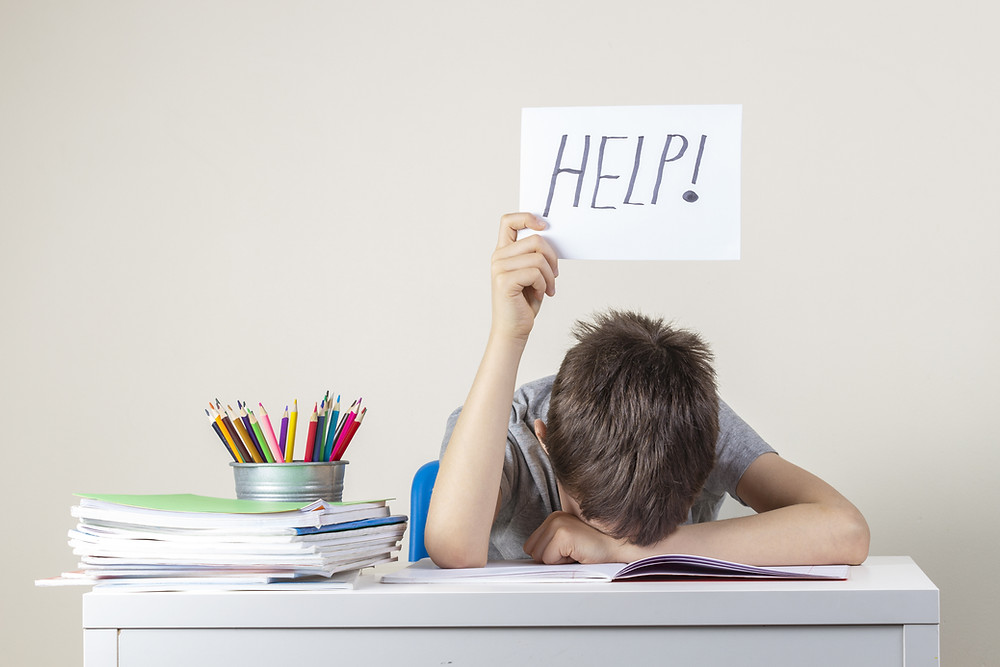Search
• by Blaine Helwig

# Why Students can’t Problem Solve without Numeracy?

The goal of modern day math classrooms is to equip students to solve everyday math problems.

There are lots of approaches to developing problem solving skills. But without a foundation of numeracy, students won’t be able to reach their full problem-solving potential. Good problem solving is dependent on students’ possessing core numeracy skills.In a way, numerical fluency is like reading fluency. Both are the building blocks of success in reading or mathematics. Reading Fluency – being able to decode words – impacts reading comprehension. Many language arts teachers have observed that students with poor phonetic decoding and sight word recognition skills read so slowly that many times they are unable to understand the very sentence they just read.

Poor numerical fluency has the same negative effect in mathematics. When a child’s numerical ability is lacking, they struggle to solve multi-part math problems. Numerical fluency is the ability to work fluidly with numbers in a variety of mathematical situations. Kids competent with numeracy possess the ability to hold a multi-part problem in their working memory, use their math skills to resolve each part and solve the problem as a whole.

Numerate students are capable of solving multi-step math problems – and importantly – evaluating the reasonableness of the solution.

For example:

Dustin saves pictures in a 4 page photo album. He has a total of 102 pictures

in the album. There are 28 pictures on the first page and there are 23 pictures

on each of the last two pages. How many pictures are on the second page of

Dustin’s photo album?

The numeracy math skills needed to solve this problem are the following:

• The ability to double (or multiply by 2): 23 x 2 = 46 pictures

• Summing two two-digit numbers with carrying: 46 + 28 = 74 pictures on three pages

• Subtracting with regrouping: 102 – 74 = 28 pictures on 4th page

• Evaluating the reasonableness of the solution (adding all the pictures together on all four pages to verify a sum of 102 pictures)If your students struggle with one of these math skills, they will be incapable – or extremely slow – to solve the problem as a whole. Most standardized tests are timed these days – so speed matters.

So what’s a school to do? Be sure to put a portion of the core math lesson each day reinforcing fundamental numeracy skills. Implementation should begin in the primary grades so students may draw upon rudimentary skills to solve the word problems they tackle in the intermediate grades.

A little investment in numeracy goes along way. Five to ten minutes of a structure makes a tremendous difference in students' mathematical abilities.